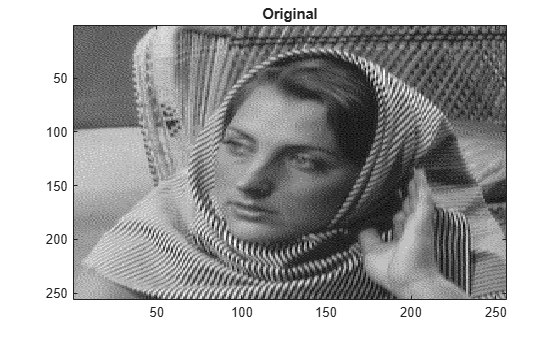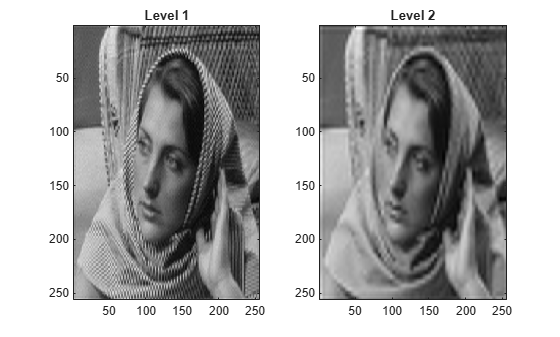# wrcoef2

Reconstruct single branch from 2-D wavelet coefficients

## Syntax

``x = wrcoef2(type,c,s,wname)``
``x = wrcoef2(type,c,s,LoR,HiR)``
``x = wrcoef2(___,n)``

## Description

`wrcoef2` is a two-dimensional wavelet analysis function. `wrcoef2` reconstructs the coefficients of an image.

````x = wrcoef2(type,c,s,wname)` returns the matrix of reconstructed coefficients of type `type` based on the wavelet decomposition structure `[c,s]` of an image (see `wavedec2` for more information) using the wavelet specified by `wname`. The coefficients at the maximum decomposition level are reconstructed. The size of `x` is equal to the size of the original image.```
````x = wrcoef2(type,c,s,LoR,HiR)` uses the lowpass and highpass reconstruction filters `LoR` and `HiR`, respectively.```

example

````x = wrcoef2(___,n)` reconstructs the coefficients at level `n` using any of the previous syntaxes.```

## Examples

collapse all

Save the current extension mode. Load an image.

```origMode = dwtmode("status","nodisp"); load woman imagesc(X) title("Original") colormap gray```Use `dwtmode` to change the extension mode to zero-padding. Obtain the 2-level wavelet decomposition of the image using the `sym5` wavelet.

```dwtmode("zpd","nodisp") [c,s] = wavedec2(X,2,"sym5");```

Reconstruct the approximation coefficients at levels 1 and 2. Display the results.

```a1 = wrcoef2("a",c,s,"sym5",1); a2 = wrcoef2("a",c,s,"sym5",2); subplot(1,2,1) imagesc(a1) title("Level 1") subplot(1,2,2) imagesc(a2) title("Level 2") colormap gray```Reconstruct the horizontal, vertical, and diagonal detail coefficients at level 2.

```h2 = wrcoef2("h",c,s,"sym5",2); v2 = wrcoef2("v",c,s,"sym5",2); d2 = wrcoef2("d",c,s,"sym5",2);```

Confirm all the reconstructions are the same size as the original image.

```sX = size(X); sa1 = size(a1); sa2 = size(a2); sh2 = size(h2); sv2 = size(v2); sd2 = size(d2); [sX;sa1;sa2;sh2;sv2;sd2]```
```ans = 6×2 256 256 256 256 256 256 256 256 256 256 256 256 ```

Restore the extension mode to the original setting.

`dwtmode(origMode,"nodisp")`

## Input Arguments

collapse all

Coefficients to reconstruct, specified as follows:

• `"a"` — Approximation coefficients

• `"h"` — Horizontal detail coefficients

• `"v"` — Vertical detail coefficients

• `"d"` — Diagonal detail coefficients

Data Types: `string` | `char`

Wavelet decomposition vector, specified as a real-valued vector. The vector `c` contains the approximation and detail coefficients organized by level. The bookkeeping matrix `s` is used to parse `c`. See `wavedec2`.

Data Types: `double`

Bookkeeping matrix, specified as an integer-valued matrix. The matrix `s` contains the dimensions of the wavelet coefficients by level and is used to parse the wavelet decomposition vector `c`. See `wavedec2`.

Data Types: `double`

Wavelet, specified as a character vector or string scalar. `wrcoef2` supports only Type 1 (orthogonal) or Type 2 (biorthogonal) wavelets. See `wfilters` for a list of orthogonal and biorthogonal wavelets.

Wavelet reconstruction filters, specified as a pair of even-length real-valued vectors. `LoR` is the lowpass reconstruction filter, and `HiR` is the highpass reconstruction filter. The lengths of `LoR` and `HiR` must be equal. See `wfilters` for additional information.

Data Types: `double`

Coefficients level, specified as an integer.

• When `type` is `"a"`, `n` must be an integer such that 0 ≤ `n``size(s,1)-2`.

• When `type` is `"h"`, `"v"`, or `"d"`, `n` must be an integer such that 1 ≤ `n``size(s,1)-2`.

Data Types: `double`

## Output Arguments

collapse all

Reconstructed coefficients, returned as a matrix. The size of `x` is equal to the size of the original image

Data Types: `double`